# Electronics and Communication Engineering - Exam Questions Papers

41.

In a JK flip-flop, J is connected to Q and K is connected to Q outputs. The JK FF converts into a

 A. RS FF B. D FF C. T FF D. Clocked RS FF

Answer: Option B

Explanation: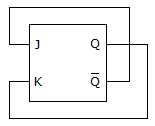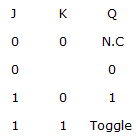Both the input is opposit to each other hence it is D FF.

42.

A point charge of 6 μC is located at the origin, uniform line charge density of 180 nC/m of 8 m length lies along the x axis and a uniform sheet of charge equal to 25 nC/m2 of area p x 42 lies in the z = 0 plane. Calculate total electric flux leaving the surface of sphere of 4 m radius centered at origin.

 A. 86.97 μC B. 8.697 nC C. 8.697 μC D. 86.97 nC

Answer: Option C

Explanation:

According to Gauss's law total flux leaving the closed surface is equal to the charge enclosed by the closed surface

Qencl = (6 x 10-6) + (8 x 180 x 10-6) + (p x 42 x 25 x 10-9)

= (6 x 10-6) + (1.44 x 10-6) + (1.257 x 10-6)

= 8.697 μ Coulombs.

43.

An infinite ladder is constructed with 1 Ω and 2 Ω resistors as shown below. Find i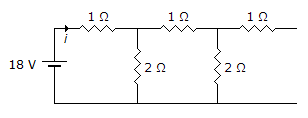A. 8.1818 A B. 0 A C. 9 A D. cannot be determined

Answer: Option C

Explanation:

As ladder is infinite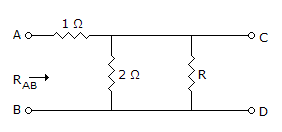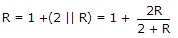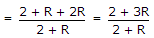2R + R2 = 2 + 3R

R2 - R - 2 = 0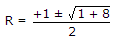R = 2 or R = -1

Negative value of R is not possible

R = 2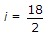i = 9 A.

44.

Consider the matrix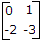The value of ep is

 A.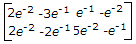B.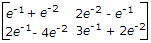C.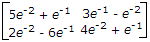D.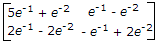Answer: Option D

Explanation: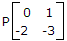find L-1[sI -A]-1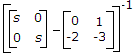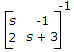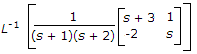find inverse Laplace transform of the expression.

45.

Find current flowing through 1 H inductor at t = 0, at which switch 'S' is opened.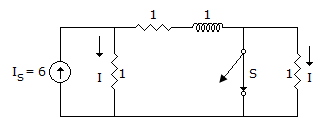A. 0 B. 2 C. 3 D. 5

Answer: Option C

Explanation: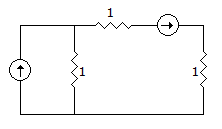For t = 0+ current in L is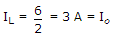circuit at t = 0+

I is Io = 3 A.

#### Current Affairs 2021

Interview Questions and Answers